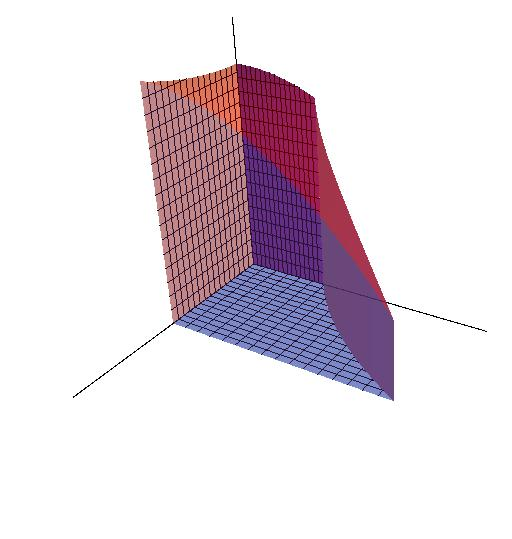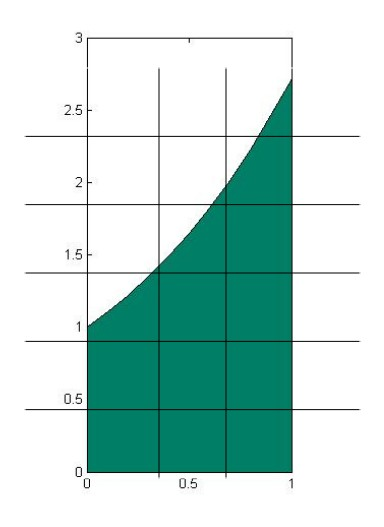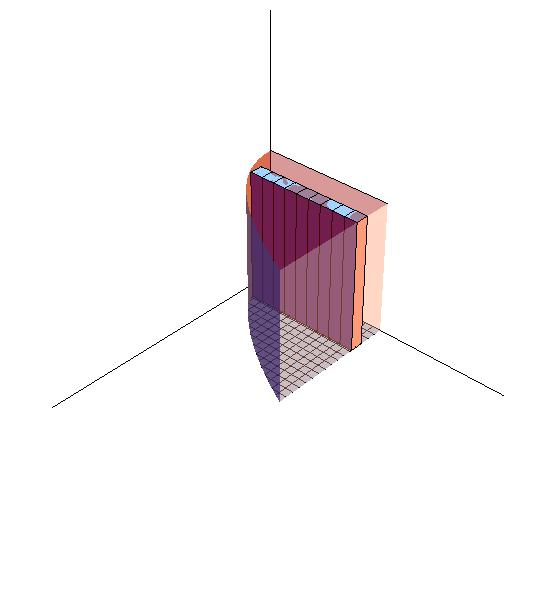$$\newcommand{\id}{\mathrm{id}}$$ $$\newcommand{\Span}{\mathrm{span}}$$ $$\newcommand{\kernel}{\mathrm{null}\,}$$ $$\newcommand{\range}{\mathrm{range}\,}$$ $$\newcommand{\RealPart}{\mathrm{Re}}$$ $$\newcommand{\ImaginaryPart}{\mathrm{Im}}$$ $$\newcommand{\Argument}{\mathrm{Arg}}$$ $$\newcommand{\norm}{\| #1 \|}$$ $$\newcommand{\inner}{\langle #1, #2 \rangle}$$ $$\newcommand{\Span}{\mathrm{span}}$$

# 3.3: Double Integrals Over General Regions

$$\newcommand{\vecs}{\overset { \rightharpoonup} {\mathbf{#1}} }$$ $$\newcommand{\vecd}{\overset{-\!-\!\rightharpoonup}{\vphantom{a}\smash {#1}}}$$$$\newcommand{\id}{\mathrm{id}}$$ $$\newcommand{\Span}{\mathrm{span}}$$ $$\newcommand{\kernel}{\mathrm{null}\,}$$ $$\newcommand{\range}{\mathrm{range}\,}$$ $$\newcommand{\RealPart}{\mathrm{Re}}$$ $$\newcommand{\ImaginaryPart}{\mathrm{Im}}$$ $$\newcommand{\Argument}{\mathrm{Arg}}$$ $$\newcommand{\norm}{\| #1 \|}$$ $$\newcommand{\inner}{\langle #1, #2 \rangle}$$ $$\newcommand{\Span}{\mathrm{span}}$$ $$\newcommand{\id}{\mathrm{id}}$$ $$\newcommand{\Span}{\mathrm{span}}$$ $$\newcommand{\kernel}{\mathrm{null}\,}$$ $$\newcommand{\range}{\mathrm{range}\,}$$ $$\newcommand{\RealPart}{\mathrm{Re}}$$ $$\newcommand{\ImaginaryPart}{\mathrm{Im}}$$ $$\newcommand{\Argument}{\mathrm{Arg}}$$ $$\newcommand{\norm}{\| #1 \|}$$ $$\newcommand{\inner}{\langle #1, #2 \rangle}$$ $$\newcommand{\Span}{\mathrm{span}}$$

In Section 15.1, we notice that all bases of the objects are rectangular. In 15.2, the area under these objects are nonrectangular. However, the method of accumulation still works.

## Introduction

In the Figure $$\PageIndex{1}$$, the green figures are the base of the 3D object on the left. Its base is encloesd by $$y=e^x$$, $$y=0$$, $$x=0$$ and $$x=1$$, and it was divided into small diamonds. As $$n$$, the number of these diamonds, goes to infinity and $$\Delta A= \Delta x \Delta y$$ goes to zero, the area of each diamond approximates to a single point, which reduces the estimation error to zero. Thus, through accumulating all these single points, we can calculate the area accurately.Figure $$\PageIndex{1}$$

$S=\lim_{n\rightarrow \infty}\sum_{k=1}^{n}\Delta A= \iint_R dA$

Theorem: Fubini's Theorem (Stronger Form)

Let $$f(x,y)$$ be continuous on a region $$R$$.

1. If R is defined by $$a \leq x \leq b, g(x_1) \leq y \leq g(x_2)$$ , with $$g_1$$ and $$g_2$$ continous on $$[a,b]$$, then

$\iint_R f(x,y) dA = \int_a^b \int_{g_1(x)}^{g_2(x)} f(x,y)\, dy\, dx$

1. If R is defined by $$c \leq y \leq d, h_1(y) \leq y \leq h_2(y)$$ , with $$h_1$$ and $$h_2$$ continous on [c,d], then

$\iint_R f(x,y) dA = \int_c^d \int_{h_1(y)}^{h_2(y)} f(x,y) \,dx \,dy$

Fubini's Theorem is usually used to calculate the volume of the objects that have nonrectangule bases.

Figure $$\PageIndex{2}$$

Just like the sample in 15.1, $$V_i = f(x,y)\Delta A$$ is the volume of the cuboid at a specific location. This cuboid has the height $$f(x,y)$$that is decided by its location. As n, the number of cuboids, goes to infinity, the shape of each cuboid approaches to a line whose height equal to $$f(x_i,y_i)$$ and error approaches to zero. Through accumulating infinity number of these "lines", we can accurately calculate the volume of the object.

$V=\lim_{n\rightarrow \infty} \sum_{k=1}^{n} f(x_i,y_i)\Delta x \Delta y = \int \int _R f(x,y)\,dA$

Example $$\PageIndex{1}$$

Now, lets calculate the volume under the surface $$f(x,y)=10+x^2-y^2$$ whose domain is enclosed by $$y=e^x$$, $$y=0$$, $$x=0$$ and $$x=1$$.Figure $$\PageIndex{3}$$

\begin{align*} &\int_0^1 \int_{y=0}^{y=e^x} 10+x^2-y^2 dy dx \\ &= \int_0^1 (10+x^2)y-\frac{1}{3}y^3 \Big|_{y=0}^{y=e^x} dx \\ &= \int_0^1 (10+x^2)e^x-\frac{1}{3}(e^x)^3 dx \\ &= 10e^x +(x^2 e^x-2xe^x+2e^x)-\frac{1}{9}(e^x)^3 \Big |_0^1 \\ &= [10e+(e-2e+2e) - \frac{1}{9}e^3]-[10+(0-0+2)-\frac{1}{9}] \\ &= 11e -\frac{1}{9} e^3 + \frac{107}{9} \end{align*}

Example $$\PageIndex{2}$$Figure $$\PageIndex{3}$$

\begin{align*} f(x,y)&=\int_{0}^{1}\int_{y=x^2}^{y=1} 1 dydx \\ &= \int_{0}^{1} y \Big|_{x^2}^{1} dx \\ &=\int_{0}^{1} 1-x^2 dx \\ &= x-\dfrac{1}{3}x^3 \Big|_0^1 \\ &= \dfrac{2}{3} \end{align*}

Reverse the order of integration of the function $f(x,y)=\int_{0}^{1}\int_{y=x^2}^{y=1}1dydx$Figure $$\PageIndex{4}$$

\begin{align*} &=\int_{y=1}^{y=0}\int_{x=0}^{x=\sqrt{y}}1 \; dx dy \\ & =\int_{0}^{1} \sqrt{y} \; dy \\ &= \dfrac{2}{3} y^{\frac{3}{2}} \Big|_{0}^{1} \\ &= \dfrac{2}{3} \end{align*}

Reminder:

1. As the equation is transformed, the domains of x and y are also transformed.
2. When $$f(x,y)=1$$, the value of double integral numerically equals to the area of its base.
3. The order of $$dx$$ and $$dy$$ decides the order of accumulation. In figure 15.2-3, stripes are arranged along y axis to form layers and then we can calculate the volume thorough adding all the layers. That's why the function is written as $$\int \int_R f(x,y)dydx$$. Similarly, in the Figure 15.2-4, stripes are arranged along x axis to form layers, so the order of dy and dx is switched. That is $$\iint_R f(x,y)dx dy$$.

Example $$\PageIndex{5}$$

Calculate the volume above $$f(x，y)=0$$ and $$f(x,y)=1$$ in the domain enclosed by $$x=\tan^{-1}(y)$$, $$x=0$$, $$y=0$$ and $$y= 1$$.

\begin{align*} f(x,y)&= \int_0^1 \int_{x=0}^{x=\tan^{-1}(y) }1 \ dx \ dy \\ &= \int_0^1 \int_{x=0}^{x=\tan^{-1}(y)} 1\ dx \ dy \\ &= \int_0^1 x \Big|_0^{\tan^{-1}} \ dy \\ &= \int_0^1 \tan^{-1}y \ dy \end{align*}

In this case, the question become too complicated to be solved. Thus, we need to transform this equation.

\begin{align*} f(x,y) &= \int_{x=0}^{x=\tan^{-1} (y)} 1 \; dx \;dy \\ &= \int_{0}^{\frac{\pi}{4}} x \Big|_{\tan (x)}^1 \;dx \\ &= \int_{0}^{\frac{\pi}{4}} (1-\tan (x)) dx \\ &= x+ \ln (\cos (x)) \Big|_0^{\frac{\pi}{4}} \\ &= (\dfrac{\pi}{4} + \ln (\dfrac{\sqrt{2}}{2}) -(0+ \ln 1) \\ &= \dfrac{\pi}{4} \ln (\dfrac{\sqrt{2}}{2}) \end{align*}

• Integrated by Justin Marshall.